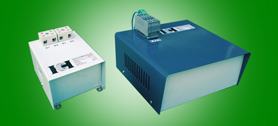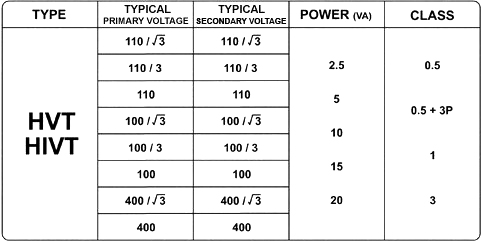###### TYPE HVT,HIVT
Harris Voltage Transformer

### Harris Voltage Transformers (VT,IVT)

The standards define a voltage transformer as one in which “the secondary voltage is substantially proportional to the primary voltage and differs in phase from it by an angle which is approximately zero for an appropriate direction of the connections.”
This, in essence, means that the voltage transformer has to be as close as possible to the “ideal” transformer. In an “ideal” transformer, the secondary voltage vector is exactly opposite and equal to the primary voltage vector, when multiplied by the turns ratio.
In a “practical” transformer, errors are introduced because some current is drawn for the magnetization of the core and because of drops in the primary and secondary windings due to leakage reactance and winding resistance. One can thus talk of a voltage error, which is the amount by which the voltage is less than the applied primary voltage, and the phase error, which is the phase angle by which the reversed secondary voltage vector is displaced from the primary voltage vector.
Harris designs its VT’s so that the resistance and reactance drops are minimized. It also uses the best grades of cold rolled grain oriented electrical steels which enables operation at optimum levels of magnetic induction, thus reducing both the size and the cost of the VT.Product List Of Type HVT,HIVT